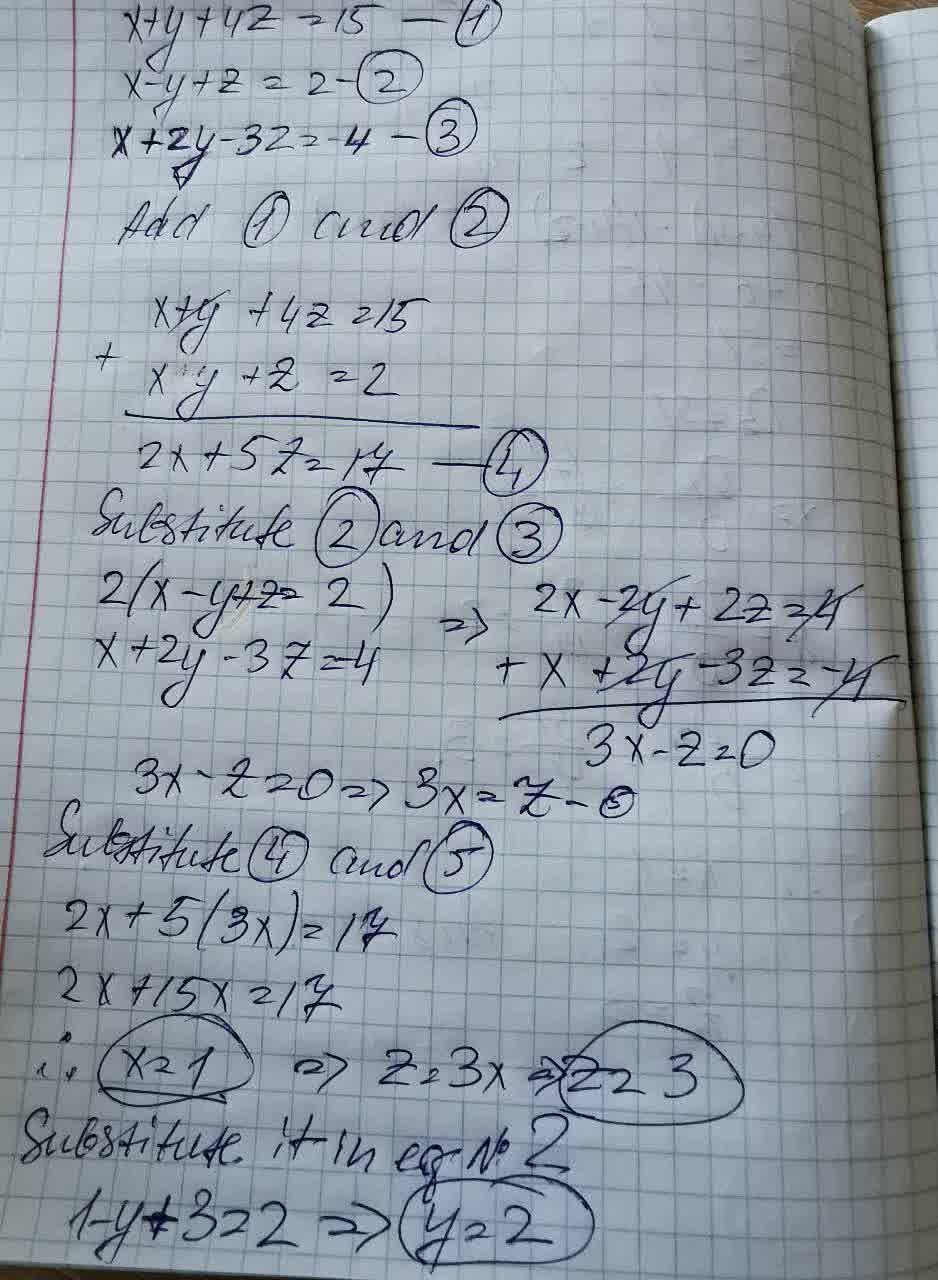# Use the elimination method to solve the following systems of linearsimultaneous equations. xty+4z=15, x-y+z=2 and x + 2y-3z=-4Jaden Easton 2021-09-03 Answered

Use the elimination method to solve the following systems of linear simultaneous equations
$$xty+4z=15$$, $$x-y+z=2$$ and $$x + 2y-3z=-4$$

• Questions are typically answered in as fast as 30 minutes

### Plainmath recommends

• Get a detailed answer even on the hardest topics.
• Ask an expert for a step-by-step guidance to learn to do it yourself.irwchh

Solving here